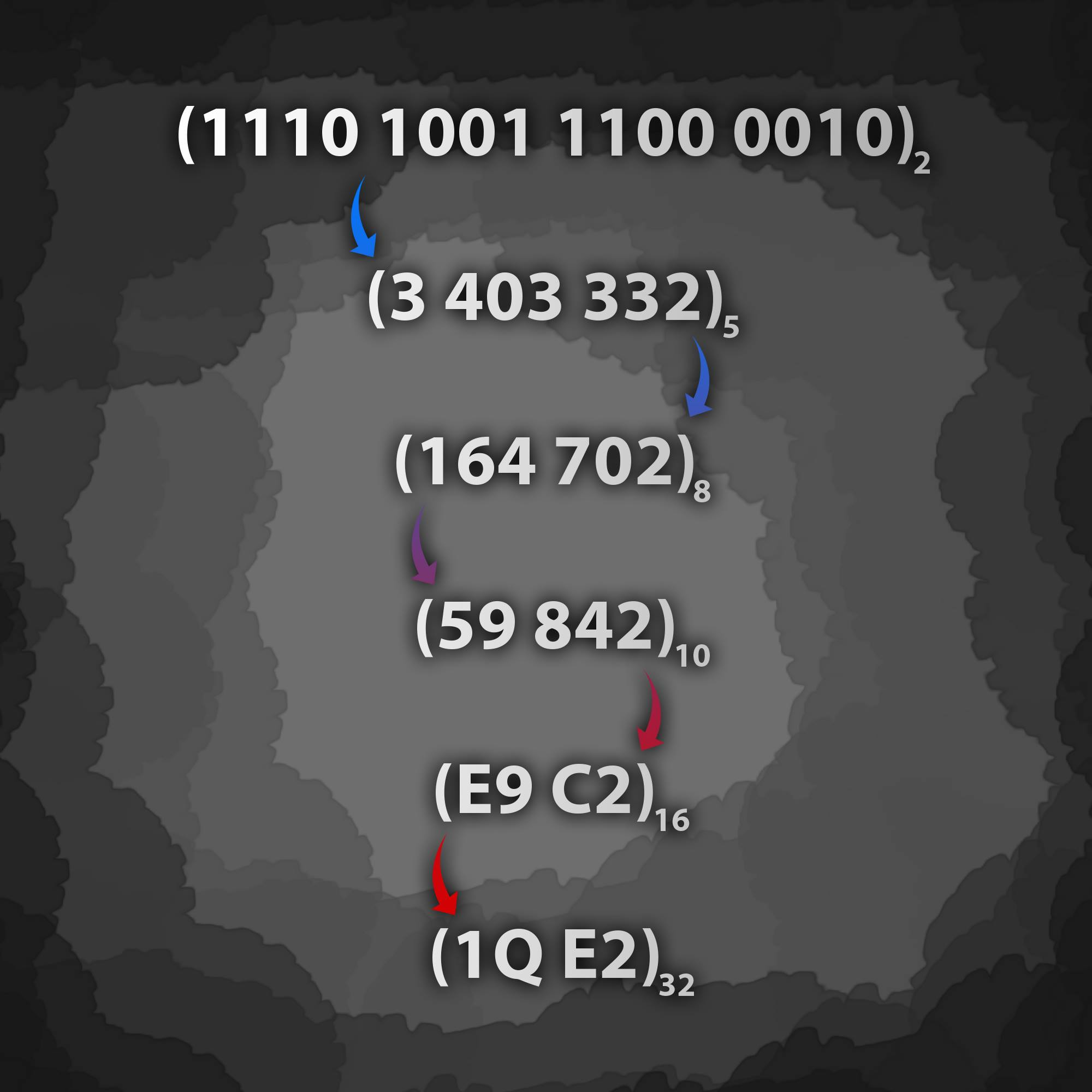# any-to-any

convert numbers between bases (any base to any base) 🔀 ..

## Usage no npm install needed!

``````<script type="module">
import anyToAny from 'https://cdn.skypack.dev/any-to-any';
</script>``````

# ANY-TO-ANY - converts numbers between bases ; binary , decimal , octal , hexadecimal and muche more ..The purpose of this module is to convert numbers from any base to other base you want.

## `Limitation`

This module can convert only the integers numbers between base 2 & base 36. Soon, it will be possible to convert real numbers [wip].

## `Installation`

``````# npm ..
\$ npm install any-to-any
# yarn ..
``````

## `Usage`

This is a practical example of how to use.

``````// const Convert = require('any-to-any')
const { Convert } = require('any-to-any')

const inputNumber  = '1110111' // 119 in decimal
const inputBase = 2
const outputBase = 8

const result = Convert(inputNumber, inputBase, outputBase)
console.log(result)
//  167

// NOTE: in case the input number is consists of numbers only,
// you can enter the number to the function in number type ..
const inputNumber  = 1110111 // 119 in decimal
const inputBase = 2
const outputBase = 8
const result = Convert(inputNumber, inputBase, outputBase);
console.log(result);
//  167
``````

NOTE: There are a set of suggested examples that have been tested that you can follow here.